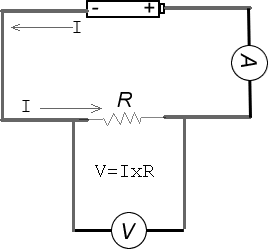#### Ohm's Law Lab

Laboratory Experiment

Introduction:

Ohm's law states that at constant temperatures the current (I) going through a load (R) is proportional to
the potential difference or Voltage (V) across it.

This law can be summarized as a simple but  very important equation:

V= I  x R

Where:
V is the voltage measured in volts,
is the current measured in amperes and
R is the resistance measured in ohms.

Purpose: To verify Ohm's Law using experimental procedures.

Materials:

• 3 resistors of different known values

• 3 batteries with holders

• Connecting wires

• Switch

• Ammeter

• Voltmeter

Procedure:

1. Connect the circuit below using the first resistor2. Have your teacher verify your circuit before you turn it on

3. Turn on the circuit and record the value of voltage and current as read by the voltmeter and ammeter respectively in the observation table below.

4. Add a second battery in series with the first and repeat step #3

5. Add a third battery in series with the first and second and repeat step #3

6. Replace the first resistor with the second and repeat steps #3 to #5

7. Replace the second resistor with the third and repeat steps #3 to #5

8. Plot a graph of voltage vs. current for each resistor on the same graph paper

9. Calculate the slope of each line (you should have three lines -- one for each resistor)

Observations Table:

 Resistor [V] Measured Voltage (Volts) [I]   Measured Current (Amps) [R] Calculated  Resistance (OHMS) 1 2 3

Analysis:

1. Write a statement to summarize your observations about the behaviour of voltage and current in an ideal resistor.

2. Write a generalized conclusion

3. Complete the following charts

a) units

 Given Rewrite these quantities using Equivalent  Unit 14,000 V KV 0.007 MB B 1.7 GHz Hz 370,000 Ω MΩ 0.007 A mA 3.30 kV V 5000 mA A 27 kΩ Ω

b) Using Ohm's Law

 Voltage (V) Current (I) Resistance (R) 1000 V 25 mA ? 0.01 V 45 MΩ ? 4.0 A 37 KΩ 67 V Green, blue, violet ? 750 mA 10Ω 300 V 1 kA ? ? 300 mA Brown, black, red 450 MV 1000 mA ?